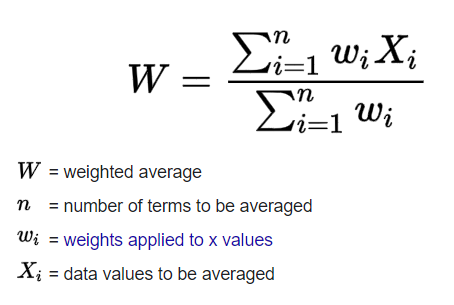Weighted average is a calculation that takes into account the varying degrees of importance of the numbers in a data set. In calculating a weighted average, each number in the data set is multiplied by a predetermined weight before the final calculation is made.

### Weighted average simple example.

For example, we have the next set of numbers: 5, 4, 3, 10, and their weights: 3, 7, 11, 4. We need to multiply the numbers and their weights among themselves and divide by the sum of the weights: (3×5+7×4+11×3+4×10) / (3+7+11+4) = 4.64.

### Weighted average python implementation

```def calculate_weighted_average(num_list, weighting_values_list):
x_numbers = []
for index, number in enumerate(num_list):
x_numbers.append(number * weighting_values_list[index])
weighting_average = sum(x_numbers)/sum(weighting_values_list)
return weighting_average

numbers = [5, 4, 3, 10]
weighting_values = [3, 7, 11, 4]

print(f"Numbers: {numbers}")
print(f"Weights: {weighting_values}")
print(f"Weighted average: {calculate_weighted_average(num_list=numbers, weighting_values_list=weighting_values)}\n")

numbers = [82, 90, 76]
weighting_values = [20, 35, 45]

print(f"Numbers: {numbers}")
print(f"Weights: {weighting_values}")
print(f"Weighted average: {calculate_weighted_average(num_list=numbers, weighting_values_list=weighting_values)}\n")
```

Output:

```Numbers: [5, 4, 3, 10]
Weights: [3, 7, 11, 4]
Weighted average: 4.64

Numbers: [82, 90, 76]
Weights: [20, 35, 45]
Weighted average: 82.1
```